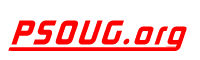Quick Search: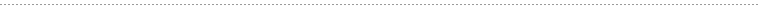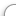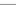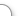CODE Oracle PL/SQL Code Library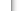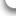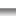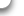JOBS Find Or Post Oracle JobsFORUM Oracle Discussion & ChatThe Oracle PL/SQL PERCENTILE_CONT Function      [Return To Index] Jump to: Select a Location PSOUG Home Page Oracle Code Library mySQL Code Library PHP Code Library JavaScript Code Library Oracle Terms & Definitions Oracle Error Codes PSOUG Community Blogs Oracle Jobs Board PSOUG Forum Oracle User Group Directory Free Oracle Magazines Online Learning Center PSOUG Presentations Advanced Code Search News and Events Sponsors Page Submit Code Contact UsLooking for the original pages? (formerly called "Morgan's Library") You can find them here.# Term: PERCENTILE_CONT

Definition:
The Oracle PL/SQL function PERCENTILE_CONT is a built in inverse distribution analytic function which works on a continuous distribution model to get the median of a column value. It takes a numeric input which is assumed as a percentile rank and a grouping specification as input. The method of linear interpolation is as below.

1. Calculate Row Number (R) of each row within the group using percentile value
2. Get CEIL(C) and FLOOR(F) value for the row number
3. If (C=F=R) then value at R
4. Else, (C-R) * (Value at F) + (R-F) * (Value at C)

Example Syntax:

```PERCENTILE_CONT() WITHIN GROUP (ORDER BY   NULLS )
OVER (); ```

Example Usage:

The SQL statement below lists the median salary which has a percentile of 0.5 within each department:

```SQL> SELECT EMPNO, DEPTNO, SALARY , PERCENTILE_CONT(0.5)
WITHIN GROUP (ORDER_BY SALARY DESC)
OVER (PARTITION BY DEPTNO) "Percentile_Cont"
FROM EMPLOYEE```